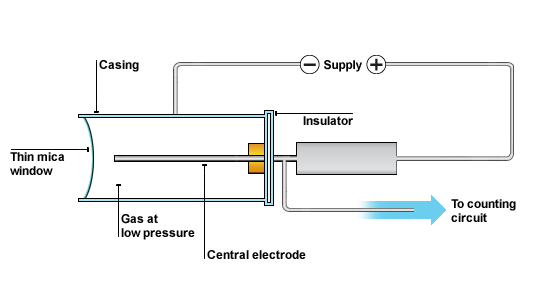• SECTION 01

(a) What is meant by radioactive decay?

Is the spontaneous disintegration of an atom by loss of sub atomic particles such as protons and neutrons which is associated with emission electromagnetic waves.

• SECTION 02

(b) A certain sample with half-life of 8 days contains 16 g of iodine 131.
(i)Write an expression to show the decay process of the sample.

Starting with the general equation of disintegration …

N = N(1/2)t/T

N – Number/Mass of atoms remained atoms at any instance

N– Initial Number/Mass of atoms at the beginning

t – Time taken from the beginning of the disintegration process

T – Time taken to reach the half-life of the material

Given No and T let us substitute to obtain a simpler expression

N = 16(1/2)t/8

(ii) Use an expression in (b) (i) to sketch the graph then estimate the mass of sample which will remain undecayed after 20 days.

From the above equation we can obtain the following values if we intuitively substitute the multiples of 8, such as t=16, t= 24, t = 32, etc…

N = 16(1/2)t/8

at t = 0 days, N = No = 16g

at t = 8 days, N = 8g

at t = 16 days, N = 4g

at t = 24 days, N = 2g

at t = 32 days, N = 1g

Plot the graph …• SECTION 03

(c) Describe the use of Geiger-Muller (G-M) tube in detecting nuclear radiations.

The GM tube is a hollow cylinder filled with a gas at low pressure. The tube has a thin window made of mica at one end. The central electrode inside the GM tube is connected to high voltage across the casing of the tube as shown in the following diagram. When alpha, beta or gamma radiation enters the tube it produces ions in the gas as they collide with gas molecules. The ions created in the gas enable the tube to conduct for a particular short time interval and consequently produce voltage pulse. Each voltage pulse corresponds to single ionizing radiation entering the GM tube. The voltage pulse is amplified and counted on the display.Geiger-Muller tube